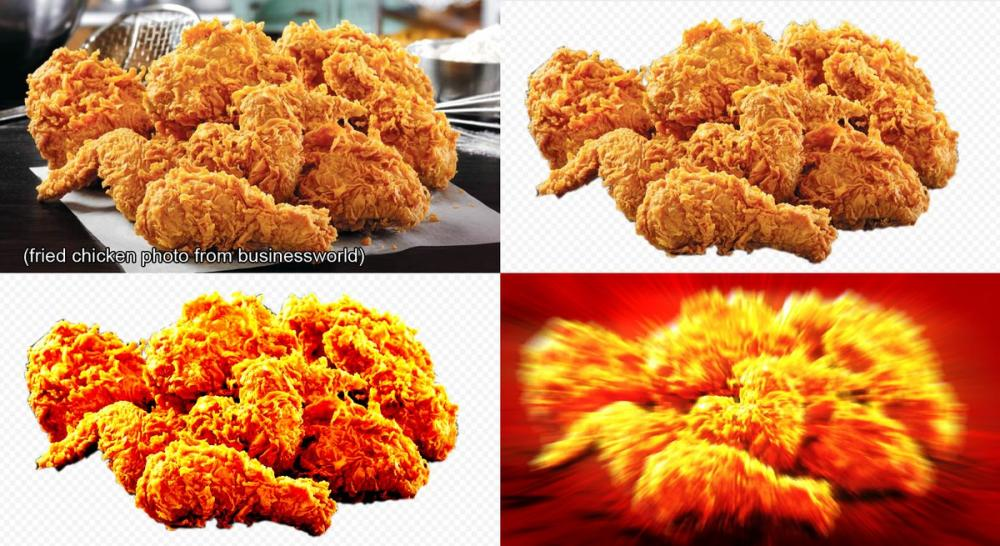## Recommended Posts

pyro solver and smoke object generate smoke and fire,
but this default setting simular result by every one.

if you want to use new noise turbulence or new shape desined noise,
use micro solver in "Velocity-update" or "Acvection"?
which solver uing?
example, spheric or box pattern.

thanks.

##### Share on other sites

sorry my poor english
Simply say.
for smoke motion, what if you want to use a completely different pattern as turbulence?
This is not necessarily a noise pattern.
It may be a forced straight line or a box-like pattern.
In such a case, other software will apply an arbitrary 3D map, but what is the similar method in Houdini?

##### Share on other sites
Posted (edited)

Disable Turbulence on shape tab.

Add GasOpenCL, connect to Velocity Update and change the code.

```#include <xnoise.h>
#include <interpolate.h>

kernel void
gasturbulence(
int                stride_x,
int                stride_y,
int                stride_z,
int                stride_offset,
float              orig_x,
float              orig_y,
float              orig_z,
float              voxelsize_x,
float              voxelsize_y,
float              voxelsize_z,
float              timeinc,
float              time,
global const void  *theXNoise,
float              amp,
float              rough,
float4             freq,
float4             offset,
float              atten,
int                turb,
int                use_control_field,
float              control_min,
float              control_max,
float              control_threshold,
float              control_influence,
int                remap_control_field,
int                controlramp_size,
constant float     *controlramp_vals,
global float       *vel_x,
global float       *vel_y,
global float       *vel_z,
global const float *density,
global const float *forcescale
)
{
size_t x = get_global_id(0);
size_t y = get_global_id(1);
size_t z = get_global_id(2);

size_t idx = stride_offset + x * stride_x + y * stride_y + z * stride_z;

// Voxel position in in local space.
float3 voxpos = (float3)(orig_x + (x + 0.5f) * voxelsize_x,
orig_y + (y + 0.5f) * voxelsize_y,
orig_z + (z + 0.5f) * voxelsize_z);
// 4D position
float4 P = (float4)(voxpos, time);
float3 v = 0;
if (density[idx] > control_threshold)
{
float scale = 1;

if (use_control_field)
{
// Fit to 0-1.
scale = fitTo01(forcescale[idx], control_min, control_max);

if (remap_control_field)
scale = lerpConstant(controlramp_vals, controlramp_size, scale);

scale += (1 - scale) * (1 - control_influence);
}

P *= freq;
P -= offset;
// The call to vop_simplexCurlNoiseVP in vop_curlNoiseVP multiplies
// roughness by 2 (maybe to match original finite differencing scheme?)
rough *= 2;
for (int i = 0; i < turb; i++, P *= 2.0f, scale *= rough)
v += scale * curlxnoise4(theXNoise, P);

if (atten != 1)
v = pow(v, atten);
v *= amp * (timeinc * 24);
}
vel_x[idx] = v.x;
vel_y[idx] = v.y;
vel_z[idx] = v.z;
}```

All that is really happening is a modification to the velocity field.

Edited by Atom

##### Share on other sites

you could use VOPs if you don't like code
https://www.sidefx.com/docs/houdini/nodes/vop/unifiednoise.html
for a box-like pattern, have a look inside the gasDisturb DOP node

##### Share on other sites

You could try the fried chicken approach and create velocity vectors using an AttributeFromMap.## Create an account

Register a new account